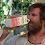## Tuesday, April 30, 2013

### Showing posterior probabilities from describe.simmap at the nodes of a plotted tree

A user comment asks the following:

"I wonder how can I alter the size of the "pie" in describe.simmap.... Same question for altering the color...."

Well, there is no way at present to change the color or pie-size in describe.simmap(...,plot=TRUE); however, describe.simmap, it is easy to recreate the posterior probability plot of describe.simmap using the function output - while customizing its attributes.

Here's a quick demo:

> # check package version
> packageVersion("phytools")
 ‘0.2.54’
>
> # first simulate some data to use in the demo
> Q<-matrix(c(-2,1,1,1,-2,1,1,1,-2),3,3)
> rownames(Q)<-colnames(Q)<-letters[1:3]
> tree<-sim.history(pbtree(n=50,scale=1),Q)
> x<-tree\$states
>
> # now the demo
> mtrees<-make.simmap(tree,x,model="ER",nsim=100)
make.simmap is sampling character histories conditioned on the transition matrix
Q =
a        b        c
a -2.041867  1.020934  1.020934
b  1.020934 -2.041867  1.020934
c  1.020934  1.020934 -2.041867
(estimated using likelihood);
and (mean) root node prior probabilities
pi =
a        b        c
0.3333333 0.3333333 0.3333333
Done.
> XX<-describe.simmap(mtrees,plot=FALSE)
100 trees with a mapped discrete character with states:
a, b, c

trees have 23.93 changes between states on average

changes are of the following types:
a,b  a,c  b,a  b,c  c,a  c,b
x->y 2.11 2.91 4.62 5.77 4.94 3.58

mean total time spent in each state is:
a        b        c    total
raw  2.2816521 4.1930936 4.2593925 10.73414
prop 0.2125603 0.3906316 0.3968081  1.00000

> hh<-max(nodeHeights(tree))*0.02 # for label offset
> plot(tree,no.margin=TRUE,label.offset=hh,edge.width=2)
> nodelabels(pie=XX\$ace,piecol=c("blue","red","green"), cex=0.5)
> tiplabels(pie=to.matrix(x,colnames(XX\$ace)), piecol=c("blue","red","green"),cex=0.5)

Obviously, to adjust the pie-sizes & colors we just change the values of cex & piecol.

That's it.

1.Great post! Very useful for plus-100-tips trees.

2.Hi, I have problems with executing describe.simmap function.
I have an object with phylogenetic trees with mapped discrete characters, but I have a problem like this:

> simPP
500 phylogenetic trees with mapped discrete characters

>describe.simmap(simPP)
Error in sort.int(x, na.last = na.last, decreasing = decreasing, ...) :
'x' must be atomic

Thank you,

1.Can you report your package version number & how the stochastic mapped trees were obtained (i.e., did they read from file or were they generated by make.simmap)?

Thanks.

3.This comment has been removed by the author.

4.Hi, Thanks for your useful post.
I want to know how to show the posterior probability of the ancestral state reconstruction at each node? Is it possible to add this information to our plot to see which of them are supported by high PP???

Thanks a lot

5.I used phytools to create stochastic character maps of a discrete character from a set of 1000 phylogenetic trees. I plot the result but I also want to see the posterior probability of nodes in a particular state. Does phytools provide that? If not, how do I plot the posterior distribution for the probability of a particular state at a particular node from the character map data itself?

Note: due to the very large amount of spam, all comments are now automatically submitted for moderation.